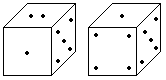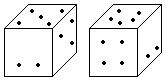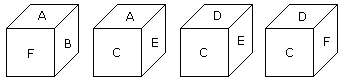# Verbal Reasoning - Dice

### Exercise :: Dice - Dice 1

16.

Observe the dots on the dice (one to six dots) in the following figures. How many dots are contained on the face opposite to the containing four dots?A. 2 B. 3 C. 5 D. 6

Explanation:

Here one of the two common faces (5) is in the same position, then according to the rule no (2) the remaining face with the 4 dots will be opposite to face with dots 2.

17.

Two positions of a dice are shown below. When 3 points are at the bottom, how many points will be at the top?A. 2 B. 5 C. 4 D. 6

Explanation:

According to the rule (2) when 3 points are at the bottom then 4 points will be at the top.

18.

From the positions of a cube are shown below, Which letter will be on the face opposite to face with 'A'?A. D B. B C. C D. F